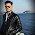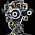## 2/09/2015

### Extracting two hog feature and comparing by vectors of descriptor in opencv (example source code)

I am wondering that two hog features can compare or not.

http://stackoverflow.com/questions/11626140/extracting-hog-features-using-opencv

I don't know this comparison is surely exact or not.

I think this comparison need more experiment.

similarity test in video

```#include < opencv2\opencv.hpp>
#include < stdio.h>

#ifdef _DEBUG
#pragma comment(lib, "opencv_core249d.lib")
#pragma comment(lib, "opencv_imgproc249d.lib")   //MAT processing
#pragma comment(lib, "opencv_highgui249d.lib")
#pragma comment(lib, "opencv_objdetect249d.lib")
#else
#pragma comment(lib, "opencv_core249.lib")
#pragma comment(lib, "opencv_imgproc249.lib")
#pragma comment(lib, "opencv_highgui249.lib")
#pragma comment(lib, "opencv_objdetect249.lib")
#endif

#define M_PI 3.1415

using namespace std;
using namespace cv;

Mat get_hogdescriptor_visual_image(Mat& origImg,
vector< float>& descriptorValues,
Size winSize,
Size cellSize,
int scaleFactor,
double viz_factor);

int main()
{

//rgb 2 gray
Mat img1_gray;
cvtColor(img1, img1_gray, CV_RGB2GRAY);

Mat img2_gray;
cvtColor(img2, img2_gray, CV_RGB2GRAY);

//resize smaller
Mat r_img1_gray;
resize(img1_gray, r_img1_gray, Size(64, 8));
Mat r_img2_gray;
resize(img2_gray, r_img2_gray, Size(64, 8));

//extractino hog feature
HOGDescriptor d1( Size(64,8), Size(8,8), Size(4,4), Size(4,4), 9);
HOGDescriptor d2( Size(64,8), Size(8,8), Size(4,4), Size(4,4), 9);
// Size(32,16), //winSize
// Size(8,8), //blocksize
// Size(4,4), //blockStride,
// Size(4,4), //cellSize,
// 9, //nbins,

//hog feature compute
vector< float> descriptorsValues1;
vector< Point> locations1;
d1.compute( r_img1_gray, descriptorsValues1, Size(0,0), Size(0,0), locations1);
vector< float> descriptorsValues2;
vector< Point> locations2;
d2.compute( r_img2_gray, descriptorsValues2, Size(0,0), Size(0,0), locations2);

//hog feature size
//cout << descriptorsValues1.size() << endl;

///////////////////////
//copy vector to mat
//create Mat
Mat A(descriptorsValues1.size(),1,CV_32FC1);
//copy vector to mat
memcpy(A.data,descriptorsValues1.data(),descriptorsValues1.size()*sizeof(float));
//create Mat
Mat B(descriptorsValues2.size(),1,CV_32FC1);
//copy vector to mat
memcpy(B.data,descriptorsValues2.data(),descriptorsValues2.size()*sizeof(float));

/////////////////////////
//sum( sqrt( (A.-B)^2 ) )
Mat C = A-B;
C = C.mul(C);
cv::sqrt(C, C);
cv::Scalar rr = cv::sum(C);
float rrr = rr(0);
cout << "Distance: " << rrr << endl;

//hog visualization
Mat r1 = get_hogdescriptor_visual_image(r_img1_gray, descriptorsValues1, Size(64,8), Size(4,4), 10, 3);
Mat r2 = get_hogdescriptor_visual_image(r_img2_gray, descriptorsValues2, Size(64,8), Size(4,4), 10, 3);

imshow("hog visualization1", r1);
imshow("hog visualization2", r2);

waitKey(0);

return 0;
}

```

1.Hello,
I am using this program to extract the features from facial images, in my case I stop to line 82, can fit these features extracted to train the SVM? Thank for the answer!

1.This code is to compare two hog feature.
Anyway, if you fail your image to extract hog feature, check grayscale? size? and so...
I posted about SVM + HOG here -> http://study.marearts.com/search/label/HOG%20feature

If you don't solve your problem keep, show your code and input image.
I will review.

Thank you.

2.Can you explain for me why is the magnitude of the gradients in the cell of the corner is similar to the magnitude of the cell at the feature image? I test your code and it work but I do not see the different magnitude at the object and the background?
One more question, when I use the image with the different size (ex 400x600) with standard parameter of HOG, why is the descriptorsValues.size is very big more than theory of HOG?
Thank you so much

1.The visualization is expressed normalized to look good.
The actual value is more complex and can not be represented visually.
http://study.marearts.com/2015/02/opencv-hog-descriptor-computation-and.html

2.I also read this thread and I run it is good. But can you explain for me how to suppressed the gradient of the background to visualize only the gradient of the object like the image of this website:
http://scikit-image.org/docs/dev/auto_examples/plot_hog.html

Because when I visualize the HOG with your code, it is still have lots of gradient of the background and it is hard to see the objects like the link above
Thank you!

3.The following link show that HOG of human image does not visualize the human object if I show it on the black screen
http://www.juergenwiki.de/work/wiki/doku.php?id=public:hog_descriptor_computation_and_visualization